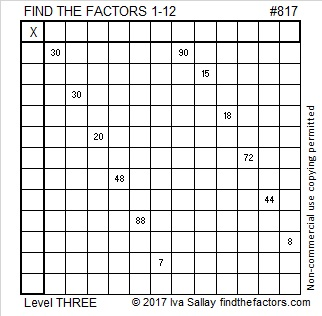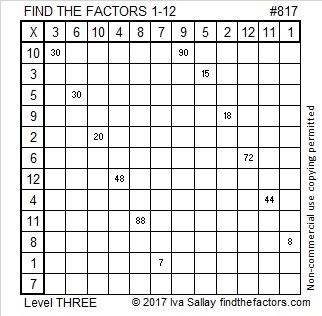# 817 Tiny Squares

Start at the top row of this level three puzzle and work down one cell at a time until you’ve written each number from 1 through 12 in both the top row and the first column. You will have solved the puzzle if all the clues given in the puzzle are the products of the numbers you wrote. You can do this!Print the puzzles or type the solution on this excel file: 12 factors 815-820

• 817 is a composite number.
• Prime factorization: 817 = 19 x 43
• The exponents in the prime factorization are 1 and 1. Adding one to each and multiplying we get (1 + 1)(1 + 1) = 2 x 2 = 4. Therefore 817 has exactly 4 factors.
• Factors of 817: 1, 19, 43, 817
• Factor pairs: 817 = 1 x 817 or 19 x 43
• 817 has no square factors that allow its square root to be simplified. √817 ≈ 28.58321817 is the sum of three consecutive prime numbers:

• 269 + 271 + 277 = 817

Because it has two factor pairs in which the numbers in the pair are either both odd or both even, 817 can be written as the difference of two squares two different ways:

• 817 = 31² – 12², which I’ve illustrated below.
• 817 = 409² – 408²Being able to be written as the difference of two squares means that 817 is a leg in two Pythagorean triples so:

• 744² + 817² = 1105²
• 817² + 333744² = 333745²

Last, but certainly not least, 817 is the difference of two consecutive cubes, namely 17³ – 16³ = 817. That means that 817 is the 17th centered hexagonal number as well! There are 17 squares on each side of the figure below and 817 tiny purple squares in all. The horizontal row in the middle has 2(17) – 1 = 33 tiny purple squares.

2(17 + 18 + 19 + 20 + 21 + 22 + 23 + 24 + 25 + 26 + 27 + 28 + 29 + 30 + 31 + 32) + 33 = 817.That difference of two cubes also means that

• 817 = 17³ – 16³ = (17 – 16)(17² + 16·17 + 16²) = (1)(289 + 272 + 256) = 817.

That’s from a³ – b³ = (a – b)(a² + ab + b²) and is illustrated below using 2D cubes instead of squares. In 3D, when the sides are folded up, the darker cubes can be claimed by two different sides so the figure will look like a 17 x 17 x 17 inside corner such as in a room where three perpendicular lines meet, but in 2D it’s just the flat net you see here:This site uses Akismet to reduce spam. Learn how your comment data is processed.